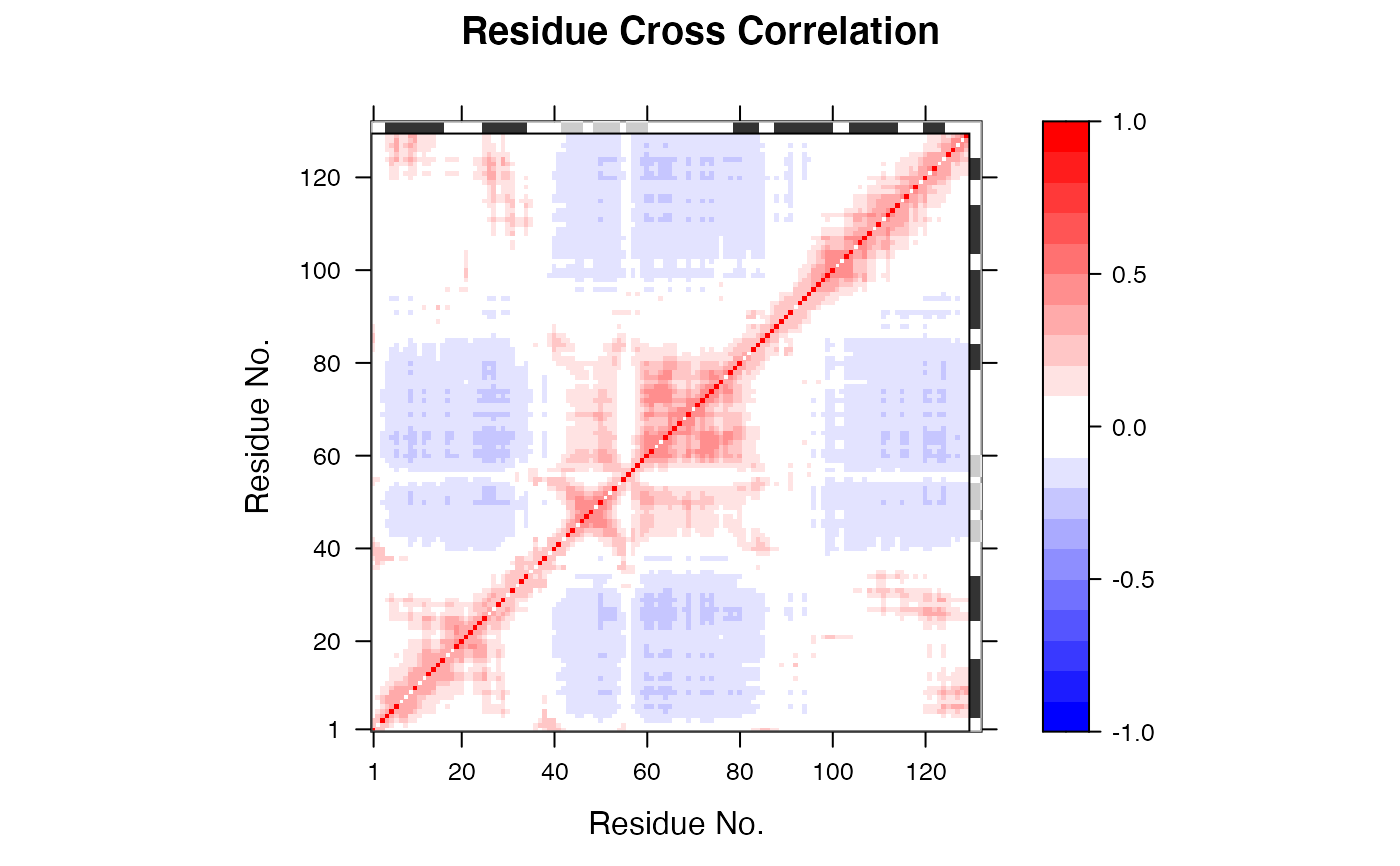Calculate the cross-correlation matrix from Gaussian network model normal modes analysis.

# S3 method for gnm
dccm(x, ...)

# S3 method for egnm
dccm(x, ...)

## Arguments

x an object of class ‘gnm’ or ‘egnm’ as obtained from gnm. additional arguments (currently ignored).

## Value

Returns a cross-correlation matrix.

## Details

This function calculates the cross-correlation matrix from Gaussian network model (GNM) normal modes analysis (NMA) obtained from gnm. It returns a matrix of residue-wise cross-correlations whose elements, Cij, may be displayed in a graphical representation frequently termed a dynamical cross-correlation map, or DCCM. (See more details in help(dccm.nma)).

## Author

Xin-Qiu Yao & Lars Skjaerven

Bahar, I. et al. (1997) Folding Des. 2, 173.

gnm, dccm.nma, dccm.enma, plot.dccm.

## Examples

## Fetch stucture
pdb <- read.pdb( system.file("examples/1hel.pdb", package="bio3d") )

## Calculate normal modes
modes <- gnm(pdb)

## Calculate correlation matrix
cm <- dccm(modes)

## Plot correlation map
plot(cm, sse = pdb, contour = FALSE, col.regions = bwr.colors(20),
at = seq(-1, 1, 0.1))